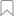## Sentiment AnalysisFREEMIUM
Verified
By Twinword, Inc. | Updated a year ago | Text Analysis
Popularity

Latency

Service Level

## 100%

Followers: 1,784
Resources:
API Creator:Twinword, Inc.
twinword
Rate API:

Is this comment positive or negative? Find out the tone.

## Sentiment Analysis

Is this comment positive or negative? Find out the tone of a user comment or post.## Interpreting the Score and Ratio of Sentiment Analysis

Our Sentiment Analysis API demo is a good place to try out our text analysis API’s ability to find the tone of a sentence or paragraph. This is useful if you have a large amount of user responses or reviews and you want to quickly find the negative comments to see what your customers don’t like and vice versa.

However, our API gives you more power than that. Besides just reading the type response (‘negative’, ‘neutral’, or ‘positive’), you can actually determine for yourself what you consider positive or negative. Below we will explain two useful information, score and ratio, that our API returns for each text analyzed.

## SCORE

The score indicates how negative or positive the overall text analyzed is. Anything below a score of -0.05 we tag as negative and anything above 0.05 we tag as positive. Anything in between inclusively, we tag as neutral.

Since we have provided the actual scores we used determine the type, you are free to reject our interpretation and interpret the actual scores yourself and create your own negative and positive minimum scores.

## RATIO

The ratio is the combined total score of negative words compared to the combined total score of positive words, ranging from -1 to 1. Take the below for example.

• Let’s say we are analyzing words A, B, C and D.

• Scenario 1) If words A and B are negative words having a combined total score of -5.0 and words C and D are positive words having a combined total score of 5.0, then the ratio would be 0 (or 1:1) as both sides are balanced.

• Scenario 2) If negative words have a total combined score of -5.0 while positive words have a total combined score of 2.5, then the ratio would be -0.667 (or 2:1).

• Scenario 3) If negative words total -5.0 and positive words total 0.0, then the ratio would be -1 (or 1:0).

In the end, the score and ratio allows you to go beyond what we think is negative or what we think is positive. In your code, you can consider the impact of the score or the impact of the ratio, or even combine them all to set your own positive and negative thresholds to suit your application.

Maybe your users are generally very negative? You can, for example, instead of using our interpretation that less than -0.05 is negative and more than 0.05 is positive, you can write your own algorithm that says below -0.9 is negative and above -0.8 is positive.

Take a look at our demo and try it out yourself.

## Sentiment Analysis

Is this comment positive or negative? Find out the tone of a user comment or post.## Interpreting the Score and Ratio of Sentiment Analysis

Our Sentiment Analysis API demo is a good place to try out our text analysis API’s ability to find the tone of a sentence or paragraph. This is useful if you have a large amount of user responses or reviews and you want to quickly find the negative comments to see what your customers don’t like and vice versa.

However, our API gives you more power than that. Besides just reading the type response (‘negative’, ‘neutral’, or ‘positive’), you can actually determine for yourself what you consider positive or negative. Below we will explain two useful information, score and ratio, that our API returns for each text analyzed.

## SCORE

The score indicates how negative or positive the overall text analyzed is. Anything below a score of -0.05 we tag as negative and anything above 0.05 we tag as positive. Anything in between inclusively, we tag as neutral.

Since we have provided the actual scores we used determine the type, you are free to reject our interpretation and interpret the actual scores yourself and create your own negative and positive minimum scores.

## RATIO

The ratio is the combined total score of negative words compared to the combined total score of positive words, ranging from -1 to 1. Take the below for example.

• Let’s say we are analyzing words A, B, C and D.

• Scenario 1) If words A and B are negative words having a combined total score of -5.0 and words C and D are positive words having a combined total score of 5.0, then the ratio would be 0 (or 1:1) as both sides are balanced.

• Scenario 2) If negative words have a total combined score of -5.0 while positive words have a total combined score of 2.5, then the ratio would be -0.667 (or 2:1).

• Scenario 3) If negative words total -5.0 and positive words total 0.0, then the ratio would be -1 (or 1:0).

In the end, the score and ratio allows you to go beyond what we think is negative or what we think is positive. In your code, you can consider the impact of the score or the impact of the ratio, or even combine them all to set your own positive and negative thresholds to suit your application.

Maybe your users are generally very negative?
You can, for example, instead of using our interpretation that less than -0.05 is negative and more than 0.05 is positive, you can write your own algorithm that says below -0.9 is negative and above -0.8 is positive.

Take a look at our demo and try it out yourself.